×

Search anything:

# Topological Sort using Breadth First Search (BFS)

#### Algorithms Graph AlgorithmsIn this article, we have explored how to perform topological sort using Breadth First Search (BFS) along with an implementation. We have compared it with Topological sort using Depth First Search (DFS).

Let us consider a scenario where a university offers a bunch of courses . There are some dependent courses too.
Example : Machine Learning is dependent on Python and Calculus , CSS dependent on HTML etc.
All these dependencies can be documented into a directed graph. Now the university wants to decide which courses to offer first so that each student has the necessary prerequisite satisfied for the course .
Thus , Topological sort comes to our aid and satisfies our need .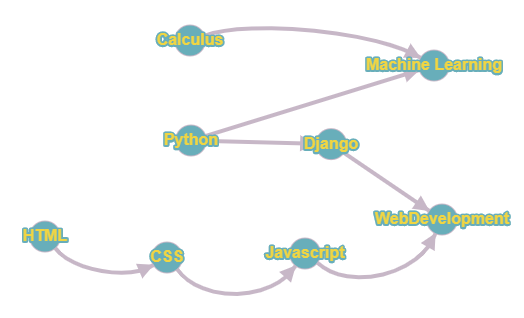All the above dependencies can be represented using a Directed Graph.

The graph in the above diagram suggests that inorder to learn ML ,Python and Calculus are a prerequisite and similarly HTML is a prerequisite for CSS and CSS for Javascript . Hence the graph represents the order in which the subjects depend on each other and the topological sort of the graph gives the order in which they must be offered to students.

Since the graph above is less complicated than what is expected in most applications it is easier to sort it topologically by-hand but complex graphs require algorithms to process them ...hence this post!!

Topological Sorting can be done by both DFS as well as BFS,this post however is concerned with the BFS approach of topological sorting popularly know as Khan's Algorithm.

## Topological Sort

Topological sorting for Directed Acyclic Graph (DAG) is a linear ordering of vertices such that for every directed edge u->v, vertex u comes before v in the ordering. Topological Sorting for a graph is not possible if the graph is not a DAG.

## Why specifically for DAG?

In order to have a topological sorting the graph must not contain any cycles.
In order to prove it, let's assume there is a cycle made of the vertices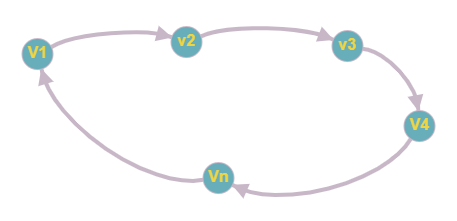v1,v2,v3,v4...vn.
That means there is a directed edge between vi and vi+1 (1<=i<n) and between vn and v1 .
So now, if we do topological sorting then vn must come before v1 because of the directed edge from vn to v1 .
Clearly, vi+1 will come after vi , because of the directed edge from vi+1 to vi , that means v1 must come before vn .

Well, this is a contradiction, here. So topological sorting can be achieved for only directed and acyclic graphs .

## Kahn's algorithm

Let's see how we can find a topological sorting in a graph.
The algorithm is as follows :

• Step1: Create an adjacency list called graph
• Step2: Call the topological_sorting() function
• Step2.1: Create a queue and an array called indegree[]
• Step2.2: Calculate the indegree of all vertices by traversing over graph
• Step2.3: Enqueue all vertices with degree 0
• Step3: While the queue is not empty repeat the below steps
• Step3.1: Dequeue the element at front from the queue and push it into the solution vector
• Step3.2: Decrease the indegree of all the neighbouring vertex of currently dequed element ,if indegree of any neigbouring vertex becomes 0 enqueue it.
• Step3.3: Enqueue all vertices with degree 0.
• Step4: If the queue becomes empty return the solution vector.
• Step5: Atlast after return from the topological_sorting() function, print contents of returned vector.

The C++ code using a BFS traversal is given below:

## C++ Implementation:

``````  vector<int> topological_sorting(vector<vector<int>> graph) {
vector <int> indegree(graph.size(), 0);
queue<int> q;
vector<int> solution;

for(int i = 0; i < graph.size(); i++) {
for(int j = 0; j < graph[i].size(); j++)
{
//iterate over all edges
indegree[ graph[i][j] ]++;
}
}

//enqueue all nodes with indegree 0
for(int i = 0; i < graph.size(); i++)
{
if(indegree[i] == 0) {
q.push(i);
}
}

//remove one node after the other
while(q.size() > 0) {
int currentNode = q.front();
q.pop();
solution.push_back(currentNode);
for(int j = 0; j < graph[currentNode].size(); j++)
{
//remove all edges
int newNode = graph[currentNode][j];
indegree[newNode]--;
if(indegree[newNode] == 0)
{
//target node has now no more incoming edges
q.push(newNode);
}
}
}

return solution;
}
int main()
{

int n,v1,v2;
cin>>n;
vector<vector<int>> graph;
for(int i=1;i<=n;i++)
{
cin>>v1>>v2;
}

cout << " Topological Sort of the given graph \n";
g.topologicalSort();

return 0;
}
``````

Let us apply the above algorithm on the following graph: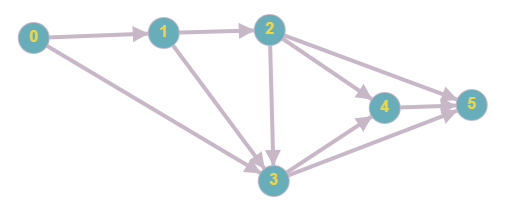Step1
Initially indegree=0 and "solution" is empty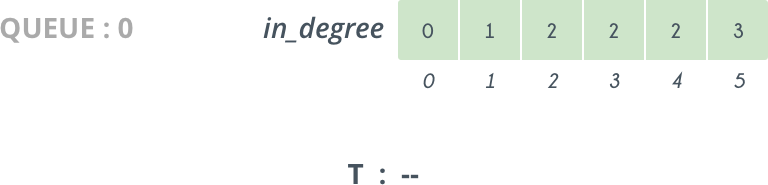Step2
So, we delete 0 from Queue and add it to our solution vector. The vertices directly connected to 0 are 1 and 2 so we decrease their indegree[] by 1 . So, now indegree=0 and so 1 is pushed in Queue.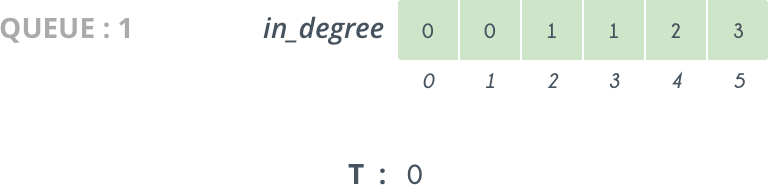Step3
Next we delete 1 from Queue and add it to our solution.By doing
this we decrease indegree by 1, and now it becomes 0 and 2 is pushed into Queue.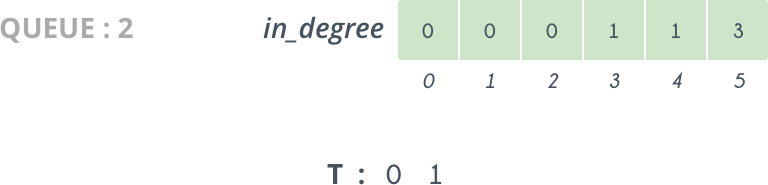Step4
So, we continue doing like this, and further iterations looks like as follows: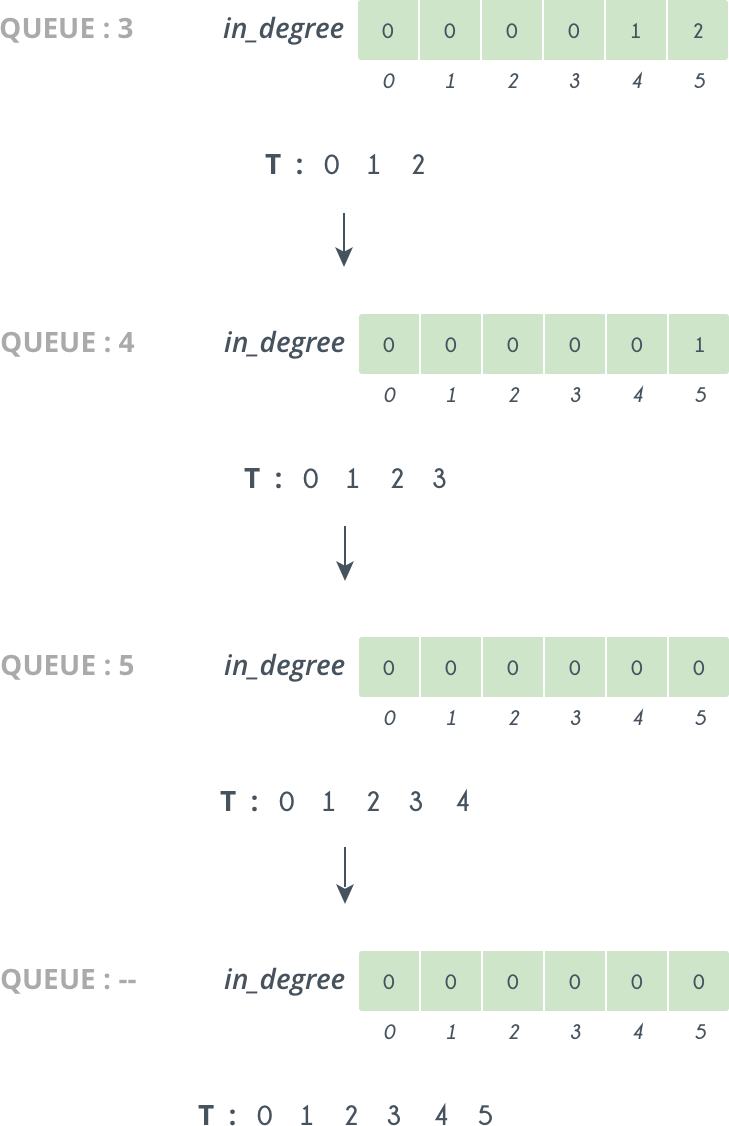So at last we get our Topological sorting in i.e. T: 0,1,2,3,4,5

## Complexity

• Worst case time complexity: `Θ(E+V)`
• Average case time complexity: `Θ(E+V)`
• Best case time complexity: `Θ(E+V)`
• Space complexity: `Θ(V)`

## DFS vs BFS

Topological sorting can be carried out using both DFS and a BFS approach .
As we know that dfs is a recursive approach , we try to find topological sorting using a recursive solution . Here we use a stack to store the elements in topological order . Using dfs we try to find the sink vertices (indegree = 0) and when found we backtrack and search for the next sink vertex.

• We can start dfs from any node and mark the node as visited.
• Perform dfs for every unvisited child for the source node.
• After traversing through every child push the node into the stack .
• After completing dfs for all the nodes pop up the node from stack and print them in the same order.
• This is our topological order for that graph.

WHY STACK?

Dfs might not produce the same result as our topological sort. Dfs prints the node as we see , meaning they have just been discovered but not yet processed ( meaning node is in visiting state ).

• For topological sort we need the order in which the nodes are completely processed .
Hence, we use stack (a natural reversing agent) to print our results in the reverse order of which they are processed thereby giving us our desired result.

This is the basic algorithm for finding Topological Sort using DFS.

1. Both DFS and BFS are two graph search techniques.
2. They find all nodes reachable.
3. They find nodes in linear time.

## Applications

• Shut down applications hosted on a server.
• Creating a course plan for college satisfying all of the prerequisites for the classes you plan to take.
• Build systems widely use this. A lot of IDEs build the dependencies first and then the dependents.

We can choose either of the appraoch as per our other needs of the question.

## Question

A very interesting followup question would be to find the lexicographically smallest topological sort using BFS!!